Trial ends in

# Liquid Phase Reactor: Sucrose Inversion

### Overview

Source: Kerry M. Dooley and Michael G. Benton, Department of Chemical Engineering, Louisiana State University, Baton Rouge, LA

Both batch and continuous flow reactors are used in catalytic reactions. Packed beds, which use solid catalysts and a continuous flow, are the most common configuration. In the absence of an extensive recycle stream, such packed bed reactors are typically modeled as "plug flow". The other most common continuous reactor is a stirred tank, which is assumed to be perfectly mixed.1 One reason for the prevalence of packed bed reactors is that, unlike most stirred tank designs, a large wall area to reactor volume ratio promotes more rapid heat transfer. For almost all reactors, heat must either be added or withdrawn to control the temperature for the desired reaction to take place.

The kinetics of catalytic reactions are often more complex than the simple 1st order, 2nd order, etc. kinetics found in textbooks. The reaction rates can also be affected by rates of mass transfer - reaction cannot take place faster than the rate at which reactants are supplied to the surface or the rate at which products are removed - and heat transfer. For these reasons, experimentation is almost always necessary to determine the reaction kinetics prior to designing large-scale equipment. In this experiment, we explore how to conduct such experiments and how to interpret them by finding a reaction rate expression and an apparent rate constant.

This experiment explores the use of a packed bed reactor to determine the kinetics of sucrose inversion. This reaction is typical of those characterized by a solid catalyst with liquid phase reactants and products.

sucrose → glucose (dextrose) + fructose(1)

A packed bed reactor will be operated at different flow rates to control the space time, which is related to residence time and is analogous to elapsed time in a batch reactor. The catalyst, a solid acid, will first be prepped by exchanging protons for any other cations present. Then, the reactor will be heated to the desired temperature (isothermal operation) with the flow of reactants. When the temperature has equilibrated, product sampling will begin. The samples will be analyzed by a polarimeter, which measures optical rotation. The mixture's optical rotation can be related to the conversion of sucrose, which can then be used in standard kinetics analyses to determine the order of the reaction, with respect to the reactant sucrose, and the apparent rate constant. The effects of fluid mechanics - no axial mixing (plug flow) vs. some axial mixing (stirred tanks in series) - on the kinetics will be analyzed as well.

### Principles

Compared to continuous stirred tank reactors (CSTRs), plug flow reactors (PFRs) are usually better suited for fast reactions and large heat effects.2 However, pressure drop and the development of "hot spots" can be problematic. Therefore, catalyst particle sizes can't be too small and careful startup procedures must be followed.

A PFR is mathematically equivalent to a large number of small, equally-sized CSTRs in series whose total volume or catalyst weight matches that of the system. When mixing occurs in the axial direction, the number of tanks, N, needed to describe reactor operation decreases. The model is called the "Tanks in Series" model. The parameters N and τ (space time) of the model can sometimes be obtained from the mean and variance of the residence time distribution (E-curve) of the reactor. For a PFR, the mean can be calculated exactly, and the variance is zero. For a real reactor, τ is usually estimated, and N is regressed from the model (Equation 2):(2)

where "i" is the reactor number, CAo is the feed concentration of the limiting reactant (in this case, sucrose), ΔfAi is the change in fractional conversion of "A" in the i the stirred tank, and rAi is the rate of reaction evaluated at the tank exit concentrations. This rate must be positive. Solving the mass balance for equal-sized CSTRs in series can also be used to determine the order of reaction for "A" using data from a real reactor and assuming the temperature can be kept reasonably constant and that N is known.

The forward rate equation for a catalytic reaction is almost always 1st order in catalyst concentration and some positive order ≤2 in any reactant concentration. Products can sometimes inhibit the catalyst, causing the reactant's order to appear less than it really is. Even reactants can inhibit the catalyst, resulting in orders for a reactant closer to zero. For these reasons, catalytic reactions are often expressed by a "power-law" model:2(3)

where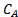is the concentration of limiting reactant, k' is the apparent rate constant, andis the apparent reaction order. This model presupposes thatthe catalyst concentration is constant (it is absorbed into the true rate constant to give k'). In real life, catalysts often deactivate, i.e., Ccat [in mmol acid sites/gcat] decreases due to accumulation of catalyst poisons. For this reason one must either account for deactivation (express Ccat as a function of time onstream) or (preferably) collect data over a time period where the catalyst is relatively stable.

The kinetics of sucrose inversion are usually found to be first order in sucrose concentration and first order in concentration of catalyst sites. Lifshutz and Dranoff report a second-order rate constant k of ~0.029 mL/(mmol acid sites • min) for a catalyst similar to the one used here at 60 °C with an activation energy of 77 kJ/mol.4 Gilliland et al. report ~1.21 mL/(mmol acid sites • min) with an activation energy of 84 kJ/mol for a similar catalyst at these conditions.4 The significant differences in k can arise from several factors: (a) effects of heat and mass transfer on the kinetics; (b) poor flow distribution; (c) poor temperature control; and (d) varying states of catalyst activation.

The space time (analogous to residence time) for a catalytic reactor is usually expressed as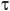= W/Q, where W is the weight of catalyst and Q is the volumetric flowrate of feed. The units on the rate constant are adjusted to account for the units on space time (i.e., for a 1st order reaction the units on k' would be the same as for 1/). Figure 1 illustrates the behavior of reactions of various kinetics orders in both a PFR and in a tanks-in-series model consisting of two tanks. Note that for positive orders, the PFR is always superior.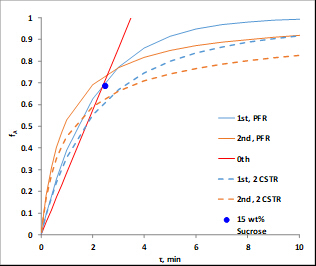Figure 1. Computed fractional conversions (vs. space time) of sucrose using rate constants derived from the data for several sucrose feeds at 60°C.

To determine the amount of sugar, a polarimeter is used, which measures the degree of rotation of the light polarized by the analyte compound. Sugars are examples of compounds with enantiomers that can be differentiated by their optical activity, the ability to rotate light. A polarimeter is especially suited to measurement of concentrations in this experiment because the reactant sucrose rotates light to the right (positive optical rotation), whereas the products glucose and fructose rotate to the left (negative optical rotation).

### Procedure

Catalyst properties are: size = 20 - 40 mesh; weight = 223 g; water content = 30 wt. %; apparent (bulk) density = 1.01 g/mL; acid site concentration = 4.6 mmol acid sites/g dry weight; surface area = 50 m2/g; macroporosity (macropore volume/total volume of cat.) = 0.34; average macropore size = 80 nm. A P&ID diagram of the unit is shown in Figure 2. For this experiment, only bed #1, the organics tank, pump and rotameter are used. Samples are collected at the upper drain. T505 is the temperature controller.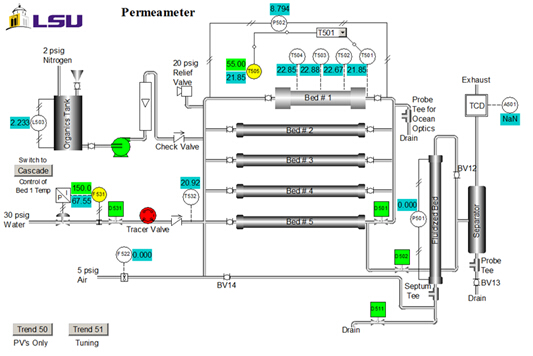Figure 2. P&ID representation of the apparatus (control system interface).

1. Starting up the Reactor

1. Access the distributed control system interface. From the Unit item on the menu, select Perm. The Perm P&ID schematic (Figure 2) will appear. All data can be collected into an Excel spreadsheet. Click "Trend 50" on the interface to obtain the key process variables with respect to time.
2. Open the inlet and exit valves to the catalytic reactor bed (Bed #1). Be sure that the inlet and exit valves to the other beds are closed, and that the control valve (F531) and on-off valve (D531) on the city water supply are also closed.
3. Add dilute acid (0.25 M H2SO4) to the feed tank (2 L).
4. Turn on the feed pump to a constant speed (at least 3 on the dial), and set the rotameter to give the desired flow rate (40 - 70 mL/min). If the rotameter cannot control flow in this range, increase the pump speed to the next higher setting.
5. Once acid has been fed, add 200 mL or more of DI water to the feed tank. The acid protons will exchange for whatever other ions (Na+, Ca2+) are attached to the sulfonic acid anions. This is because even DI water contains impurities and municipal water contains even more. This is called "regenerating" the catalyst.
6. Prepare at least 10 L of feed solution with 15 wt% sucrose in DI water. When preparing the sucrose solution, add the sucrose slowly to the water while stirring at room temperature. Use both a magnetic stirrer and a paddle.
7. Add the feed into the organics tank.

2. Packed Bed Reactor Operation

1. To begin sucrose feed flow, turn on the pump, and adjust flow as desired using the pump's speed controller and the rotameter. The speed controller is for gross adjustment, whereas the rotameter is for fine adjustment.
2. Program the setpoint for T505 to 50°C, and set it to AUTO. The system is programmed to cut off the heaters if the bed temperature reaches 90°C, or if the pressure drop, as measured by the d/p transmitter, falls below 1 in. on the H2O column pressure, which indicates that there is no feed.
3. A typical temperature for the reaction is 60°C. When the reactor temperature first exceeds 50°C, move the set point to this desired final temperature. A temperature profile may be observed across the catalyst section, which can be visualized by examining thermocouples T502 and T503.
4. Collect 25 mL samples of the reaction products at the upper drain in test tubes or sample bottles 10 min apart. Do not collect samples until at least two bed residence times (the bed open volume is ~1.2 L) have passed.

3. Shutting Down the System

1. Set the T-505, Bed #1 temperature control to zero output on MANUAL.
2. Shut off flow to the reactor once the temperature begins to decrease.
3. Close the block valves on Bed #1.

4. Using the Polarimeter

Analyze reaction product samples and the initial feed with the polarimeter. For a 15 wt. % sucrose feed, conversions should be in the range of 80 to 120 mL/min. Pure sucrose, glucose and fructose should be used as the calibration standards. See the appendix for more details on polarimetry.

1. Turn on the sodium lamp and allow it to warm up for approximately 5 to 10 min. A yellow light will be visible.
2. Check the zero position of the dial. At zero, a uniform dark field with no dark/light fringes, should be observed.
3. Add 25 mL of the initial feed solution to a clean tube. Make sure the tube is completely filled. Use the same tube for both calibrations and measurement because the reading is path length dependent.
4. Place the tube in the polarimeter. The tube should be facing up to remove any trapped air from the line of sight and the bulb should be near the eyepiece.
5. Close the cover. If the solution rotates polarized light, dark/light fringes should be observed through the lens.
6. Rotate the dial until the fringes disappear and a uniform dark field is observed.
7. Read the rotation angle through the magnifying glass using the vernier scale. The dial divisions are 1 degree each and each vernier division is 0.05°. To adjust the focus, rotate the black dial located below the eyepiece.
8. Repeat for each sample. Use DI water to clean the tube before each measurement.

Chemical reactions are carried out in various types of reactors using catalysts in order to increase reaction rate and improve conversion. Reaction rate is temperature dependent thus it is strongly influenced by heat transfer. Additionally, reaction rate is effected by mass transfer since a reaction cannot take place faster than the rate at which reactants are supplied to the catalyst surface. Therefore, packed bed reactors are often preferred over batch reactors as rapid heat transfer is more feasible. In this video, the kinetics of a simple reaction in a packed bed reactor is analyzed. The reactor is operated at different conditions in order to determine the real reaction order and a parent rate constant, as the kinetics of real systems often deviate from what is expected.

Packed bed reactors can be modeled as a series of many equally sized CSTRs who's total volume and catalyst weight matches that of the packed bed reactor. This model is called the tanks-in-series model and is given by this equation. Here, i is the reactor number, CA0 is the feed concentration of the limiting reactant, and delta FAI is the change in fractional conversion of the limiting reactant. Finally, RAI is the rate of reaction, N is the number of tanks needed, and Tao is residence time. The forward rate for a catalytic reaction is almost always first order with respect to catalyst concentration and some positive order less than two with respect to reactant concentration. However, catalyst inhibition may alter reaction order, causing the reaction order to appear less than it actually is. Even reactants can inhibit the catalyst causing the reaction order to appear close to zero. For these reasons, catalytic reactions are described by the power law model where K prime is the apparent rate constant, CA is the concentration of the limiting reactant, and beta is the apparent reaction order. The model presupposes that catalyst concentration is constant. However, in practice, catalysts deactivate. Thus catalyst concentration should be modeled as a function of time. In the following demonstration, the kinetics of a typical reaction with a solid catalyst and liquid phase reactants and products is demonstrated. The reaction involves the breakdown of sucrose into glucose and fructose called sucrose inversion. The reaction is typically first order with respect to sucrose and with respect to catalyst sites. The rate constant is effected by heat and mass transfer, flow distribution, temperature, and catalyst activation. Thus the rate constant is determined experimentally for the specific system. Now that we have discussed the tanks-in-series model and how to deduce the reaction kinetics, let's take a look at the procedure itself.

Before you start, familiarize yourself with the apparatus. Select Perm from the unit item on the menu to access the permeameter schematic. In this experiment, the unit is operated using a distributed control system. Only bed number one, the organics tank, pump, and T505 temperature controller are used. By selecting Trend 50, all the data, the key process variables with respect to time can be obtained and collected into a spreadsheet. Now, open the inlet and exit valves to catalytic reactor bed number one. Make sure that the inlet and exit values to the other beds are closed as well as the control valve F531 and the on/off valve D531 on the city water supply.

Add dilute acid to the two liter tank. Turn on the feed pump to a constant speed and set the rotameter to obtain a desired flow of 40 to 70 milliliters per minute. Increase the speed of the feed pump if the rotameter cannot reach this range of flow. Feed the acid and then approximately 200 milliliters of deionized water to regenerate the catalyst by exchanging the cations such as sodium or calcium which are interacting with sulfonic acid anions. Next, prepare the sucrose feed solution and add one liter to the organics tank. Turn on the pump. Use the speed controller of the pump and the rotameter to adjust the speed flow as desired. Set the T505 temperature controller to auto and select a set point of 50 degrees Celsius. When the system has reached 50 degrees, move the set point to the final temperature of 60 degrees where the reaction is typically carried out.

First, use a test tube to collect at least 25 milliliters of the initial feed to have a sucrose sample before the reaction has started. Then wait until two bed residence times have passed and collect two sets of 25 milliliter samples at the drain which are 10 minutes apart. These samples will be analyzed using a polarimeter. To begin reactor shutdown, set T505 to zero output. Once the temperature begins to drop, shut off the reactor and then close the block valves on bed one. Now use a polarimeter to analyze the samples. A polarimeter is used because carbohydrates are enantiomers and rotate polarized light to a certain degree. Sucrose rotates the light to the right while the solution of glucose and fructose will rotate it to the left giving negative values. Turn on the sodium lamp and wait until a yellow light is seen. A uniform dark field is visible at the zero position of the dial. Transfer 25 milliliters of the reaction sample to the tube and place it into the polarimeter with the bulb near the eye piece facing up and then close the cover. Dark and light fringes can be observed through the lens if the reaction sample rotates polarized light. Rotate the dial until the fringes disappear and reveal a uniform dark field. Adjust the focus with the black dial and using the Vernier scale, read the rotation angle through the magnifying glass to determine the fraction conversion of sucrose.

Now let's take a look at the rate constant determination using the fractional conversion of sucrose in a packed bed reactor. The specific rotation D of each sugar can be found in the literature and is correlated to the measured rotation and the concentration. Concentration is then used to determine fractional conversion. This data is shown here, plotted against the degree of rotation. The higher the concentration of sucrose, the higher the positive degree of rotation. As the reaction progresses and sucrose is converted to glucose and fructose, the positive degree of rotation diminishes. Now let's take a look at the reaction kinetics of sucrose inversion at 60 degrees Celsius. Calculate the pseudo first order constant K prime for each feed concentration, which ignores the first order dependents of the catalyst. Then account for the first order dependence of the catalyst by dividing the pseudo first order rate constant by the concentration of the catalyst to give the second order rate constant K two. To determine the real reaction order for the data acquired, start with the generalized mole balance of the packed bed reactor with respect to catalyst weight W. Then determine the equations for each reaction order. Fit these equations to the data using a non-linear regression and determine the sum of squared errors to evaluate the fit. Now fit the data to the tanks-in-series model for the first order reaction and determine the number of tanks needed. A small number of tanks is calculated suggesting that the reaction deviates from ideal packed bed reactor behavior. This is most likely attributed to axial mixing and temperature fluctuations within the reactor. Finally, we can compare the reaction behavior of various kinetic orders, including first and second order packed bed reactor models with first and second order tanks-in-series models consisting of two tanks. It is clear that the fractional conversion for the first order packed bed reactor model more closely represents the observed behavior as matched to the known data point for 15 weight percent sucrose.

Solid catalysts are used in a wide range of applications and reactor setups as they are one of the most important fields in modern technology. A fluidized bed reactor utilizes solid catalyst suspended in fluid. The fluid, usually gas or liquid, is passed through solid catalyst particles at high enough velocities to suspend them and make them behave like a fluid. These types of reactors can be used for many different applications, one of which is the pyrolysis of lignocellulosic biomass. In this process, the thermal decomposition of biomass occurs resulting in oxygenated bio oils. Catalyst performance varies depending on operating conditions, which can be measured using a temperature programmed reaction. A temperature programmed reaction involves the steady increase of reaction temperature with the continuous monitoring of reactor effluent. Performance is then correlated to temperature enabling the determination of optimum operating temperature.

You've just watched Jove's introduction to packed bed reactors for catalytic reactions. You should now understand how to analyze the kinetics of the reaction and how to model behavior using the tanks-in-series model. Thanks for watching.

### Results

The polarimeter determines the fractional conversions of sucrose after reaction in a packed bed reactor. A previous polarimeter calibration for a three different sucrose feeds is shown in Figure 3.Figure 3. Relationship between degree of rotation and fractional conversion of sucrose for different feed concentrations.

Sample data are presented in Figure 4 for the reaction at 60 °C at varying sucrose feed concentrations. Fractional conversions were calculated directly from the polarimeter calibration curve using the following equation, where D is the degrees of rotation from the polarimeter: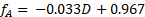(4)Figure 4. Sucrose inversion reaction at 60°C, 100 mL/min feed rate.

For both 0th and 1st order reactions, the conversion in a PFR is independent of feed concentration.2 Additionally, k' should be invariant for 1st order kinetics. Assuming the reactor to be a PFR, the 2nd order rate constant, k2 (mL/mmol sites • min), was determined by accounting for the 1st order dependence the catalyst, and the pseudo-1st order rate constant k' (mL/gcat • min) was determined by ignoring the 1st order dependence of the catalyst. The results of the pseudo-k' calculations are plotted in Figure 4. And the value of k2 was found by dividing k' by the concentration of catalyst (mmol acid sites/gcat) given previously.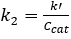(5)

To determine whether the reaction kinetics were closer to 0, 0.5, 1, 1.5 or 2nd order in sucrose, nonlinear regression of the mass balance was used, and the sum of squared errors was minimized for all three runs. In order to use nonlinear regression, an objective function was formulated based on the integrated PFR mass balance and the respective reaction order. For example, the following is the objective function for a 1.5 kinetic order in sucrose concentration:(6)

Other objective functions can be formulated from the standard PFR mass balance solutions, which can be found in all kinetics textbooks.2 The experimental data in Figure 4 were fit to the integrated PFR mass balances for 1, 1.5 and 2 orders with respect to sucrose. The sum of squared errors for the three reaction orders were determined to be 0.39, 0.16 and 1.3, respectively. Therefore, the best fit was found to be n = 1.5 order. This leads to a k' value of 35 (mL/gcat • min).

It was initially thought that the kinetics were 1st order with respect to sucrose.2-3 Using this assumption, one can determine the number of equal volume CSTRs, N, in series that are required to model this reactor. Again, the sum of squared errors in the mass balance for all three runs were minimized to determine both N and k'. The data were fit to the tanks-in-series model for 1st order reactions: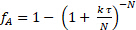(7)

It was found that N = 2.1 "tanks" and k' = 0.62 mL/gcat • min. This is not a great fit because the reaction order is not exactly 1. The data suggest a sucrose order > 1. The relative standard deviations of fA were at most 2%, which is easily accounted for by the variation in temperature (as high as 9 °C). There was no evidence of catalyst deactivation. The fractional conversions for both a PFR and for two CSTR tanks in series was computed using the k's from nonlinear regression and plotted in Figure 1. For zeroth order, there was no difference between a PFR and CSTRs in series because the rate is independent of sucrose concentration. If the curves for 6 or greater CSTRs had been plotted, they would have coincided closely with the PFR curves. The predicted fractional conversions for two CSTR tanks in series is slower than a PFR for all reaction orders. The experimental data for 15 wt% sucrose is actually closer to a first-order reaction in PFR.

The error in k' can be estimated by comparing the differences in computed k' values at the average temperature deviation (4.5 °C) to the temperature of the reaction, 60 °C, using the Arrhenius equation and averaging the two literature activation energies. The estimated k' for 1.5 order kinetics at 64.5 °C is 52 (mL/mol)0.5 mL • gcat-1 • min-1, which is almost 50% higher than the regressed value of 35 (mL/mol)0.5 mL • gcat-1 • min-1. Slight variations in temperature can affect the k' greatly.

### Applications and Summary

The reaction does not behave exactly as expected because the apparent order n is > 1. Of all the phenomena that can cause such deviations in real reactors, deviations from ideal PFR behavior caused by axial mixing are suggested by the fact that fitting to the tanks-in-series model gives only a small number of tanks - for a perfect PFR, N should be at least 6. Such deviations are often found in relatively short beds, especially if the flow is multiphase (some water is vaporized in the reactor). However, another cause of the deviation is less apparent but probably even more important. The reaction is highly exothermic, and as mentioned, the temperature oscillated by as much as 9°C (mostly above the set point). The more sucrose in the feed, the more heat that will be generated. As might be expected, the oscillations were most significant with the 20 wt% feed. This suggests another reason for an apparent order n > 1: the more heat generated at a higher concentration of feed increases the reactor temperature more, which in turn increases the reaction rate resulting in a derived apparent order > the actual order. If the temperature is inadequately controlled, the reactor temperature might increase to the adiabatic limit. Deviations from ideal PFR behavior in both flow and temperature can affect the apparent kinetics derived from actual reactors, putting a premium on careful reactor scale-up to duplicate pilot-plant conditions of fluid flow and heat transfer.

Packed bed reactors have many uses in the chemical industry. Sulfuric acid, a chemical used to make hundreds of different products, is commonly manufactured in part using packed bed chemical reactors in series. Over 200 million tons are produced annually.In this reaction, sulfur dioxide and air are passed through fixed bed reactors in series (with intermediate heat exchangers for heat removal) containing a supported vanadium oxide catalyst at high temperatures.4 The SO2 is oxidized to SO3, which, when absorbed in water, makes sulfuric acid.

A more recent use for packed bed reactors is in production of biodiesel by transesterifying triglycerides, or esterifying fatty acids, with methanol. While biodiesel is produced in different ways, packed bed reactors can be advantageous for continuous production. Biodiesel is considered a renewable energy source because it is produced from algae or waste foodstuffs, and because it is biodegradable and non-toxic. Regardless of the catalyst used, it must be thoroughly purged from the product after the reaction, because even small amounts can render the fuel unusable.5

APPENDIX A – USING THE POLARIMETER
Polarimetry measures the extent to which a substance interacts with plane polarized light (light which consists of waves that vibrate only in one plane). It can rotate the polarized light to the left, to the right, or not at all. If it rotates polarized light to the left or to the right, it is “optically active”. If a compound does not have a chiral center, it will not rotate polarized light. The number of degrees and the direction of rotation are measured to give the observed rotation. The observed rotation is corrected for the length of the cell used and the solution concentration, using the following equation: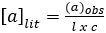(A1)
where: a = specific rotation (degrees) (literature value), l = path length (dm), and c = concentration (g/mL).
Comparing the corrected observed rotation to literature values can aid in the identification of an unknown compound. However, if the compounds are known, it is more common to prepare calibration standards of the unknowns and correlate the observed rotation to concentration.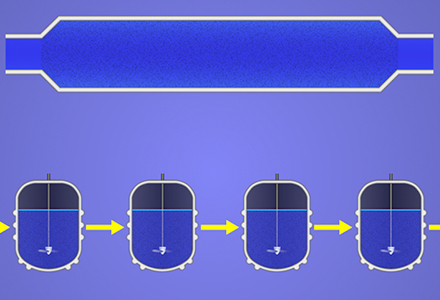Play Video

### Cite this

JoVE Science Education Database. Chemical Engineering. Liquid Phase Reactor: Sucrose Inversion. JoVE, Cambridge, MA, (2021).More

JoVE Science Education Database. Chemical Engineering. Liquid Phase Reactor: Sucrose Inversion. JoVE, Cambridge, MA, (2021).

Less
Copy Citation
View Video

### Get cutting-edge science videos from JoVE sent straight to your inbox every month.X